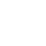# DPR Aptitude test preparatory questions

See the few questions below and use them to have an insight on what the DPR aptitude test questions might look like.Published

onPlease use this material without limiting yourself to what is herein, be wise.

1. When walking, a certain person takes 16 complete steps in 10 seconds. At this rate, how many complete steps does the person take in 72 seconds?
A. 45
B. 78
C. 86
D. 90
E. 115

2. In computer jargon, what do you understand by ROM?
B. Random Only Memory
C. Real Output Memory

3. A body is floating in seawater with 2/3 of its volume above the surface. The weight of the displaced water is?
A. Less than the weight of the body
B. Equal to the weight of the body
C. More than the weight of the body
D. Not enough information provided.

Questions 4 – 9 refer to the following: A tank is a vertical cylinder with inside diameter of 6ft and a height of 15ft.

4. What is the volume of liquid in cubic feet if we fill the tank to one foot high?
A. 28.27 ft3
B. 18.8 ft3
C. 113.1 ft3
D. 424.1 ft3.

5. What is the total volume of the tank in cubic feet?
A. 1686.5 ft3
B. 442.5 ft3
C. 424.1 ft3
D. 847.5 ft3.

6. What is the volume of water in cubic ft in the tank if a measure stick indicates the water surface is 6 feet below the top of the tank?
A. 170.2 ft3
B. 254.4 ft3
C. 1017.9 ft3
D. 678.6 ft3.

7. What is the weight in pounds of the tank, as in the above case, if the tank itself weighs 20 lbs per ft of height?
A. 63,424 lbs
B. 42,241 lbs
C. 16,151 lbs
D. 10,605 lbs.

8. How much water in gallons would have to be removed to reduce the total weight of the tank plus water, as in the above case, by 20%?
A. 388 gals
B. 3,230 gals
C. 2,121 gals
D. 626 gals.

9. What would be the new level of the water, as measured from the top of the tank, if the water in the above case (equivalent of 20% of total weight) is removed?
A. 10.4 ft
B. 12.7 ft
C. 7.1 ft
D. 7.8 ft.

10. If everybody at a party shook and with each other, and there was a total of 66 handshakes. How many people attended the party?

For questions 11 to 13, four words or phrases have been highlighted in each sentence. Please choose the one word or phrase, which is incorrect in standard written English.For questions 14 to 18, select the correct preposition or adverb to fill in the gap:

14 He insisted  _____  seeing the documents.
A. by
B. in
C. of
D. on

15.  It never occurred  _____  me to ask him for proof of his identity.
A. for
B. to
C. on
D. with

16.  I object to being kept waiting. Why can’t you be  _____  time?
A. on
B. in
C. of
D. at

17.  I was  _____  the impression that I had paid you for the work you did for me.
A. into
B. over
C. with
D. under

18.  Buying presents for children is sometimes very difficult.  ___  the end I bought a kite for Tom.
A. At
B. On
C. In
D. By.

19.  What are two safety precautions that should be taken before driving your car?

20.  In computer jargon, what do you understand by CPU?
A. Computer Processing Unit
B. Computer Procedure Utility
C. Central Processing Unit
D. Central Power Unit

21.  The five cogs are turning in the same direction and at the same speed. Which two will clash at a given moment?A. A and B
B. B and C
C. C and D
D. D and E

22.  What is the equation for Ohm’s law?
A. V = IR
B. V = I/R
C. R = VI
D. I = VR

23.  In direct current I = 3A, R = 4W. Calculate V
A. 1.33 V
B. 6 V
C. 12 V
D. 24 V

24.  What becomes V if we use 2 resistors of 4W in parallel?
A. 2.66 V
B. 6 V
C. 12 V
D. 24 V.

25.  Which of the following pair of countries consist of the permanent security council of UN
A. Brazil, Germany, France, USA, China
B. France, China, USSR, USA, Britain
C. France,Britain, Brazil, Newzealand
D. France, Germany, Japan, China

26.  In order to qualify for the post of President in Nigeria the person must be _____ and above
A. 35 years
B. 20 years
C. 40 years
D. 55 years

27.  The name Nigeria coined out of _________
A. Niger Forest
B. Niger Area
C. Niger River
D. Niger Textures

28.  The first Inspector General of the Police Force was
A. Teslim Balogun
B. Louis Edet
D. Mike Okiro.